Use Excercise 35 to show that a simple graph with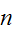vertices and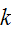connected components has at most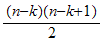edges.

Solution:

Excercise 35 proved that a simple connected graph has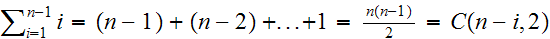edges.

A simple graph withvertices andconnected components has at leastvertices, ie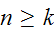.

The amount of edges in such a graph are maximized when a single component has the maximum amount of vertices and still satisfies.

In this case, every component will have only one vertex, except one, which has all of the other vertices.

All components except one will have no edges.

The other component will havevertices.

If we put this into the formula from #35, we have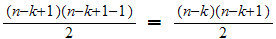edges.

This document created by Scientific Notebook 4.0.